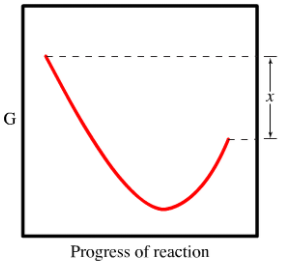# Problem: The diagram shows the free energy change of the reaction. A(g) + B(g) ⇌ AB(g) The reaction progress starts on the left with pure reactants, A and B, at 1 atm and moves to pure product, AB, also at 1 atm on the right. Select the true statements.A. The "x" on the graph corresponds to ΔG of this reaction.B. The minimum on the graph corresponds to the equilibrium position of the reaction.C. At equilibrium, all of A and B have reacted to form pure AB.D. The difference between the top left of the curve and the bottom of the curve corresponds to ΔG of this reaction.E. The entropy change for the reaction is positive.

87% (62 ratings)
###### FREE Expert Solution
• Analyzing the choices

• A. True since the change is between the starting point (our reactant) and the ending point (product)

87% (62 ratings)###### Problem Details

The diagram shows the free energy change of the reaction. A(g) + B(g) ⇌ AB(g) The reaction progress starts on the left with pure reactants, A and B, at 1 atm and moves to pure product, AB, also at 1 atm on the right. Select the true statements.

A. The "x" on the graph corresponds to ΔG of this reaction.

B. The minimum on the graph corresponds to the equilibrium position of the reaction.

C. At equilibrium, all of A and B have reacted to form pure AB.

D. The difference between the top left of the curve and the bottom of the curve corresponds to ΔG of this reaction.

E. The entropy change for the reaction is positive.What scientific concept do you need to know in order to solve this problem?

Our tutors have indicated that to solve this problem you will need to apply the Gibbs Free Energy concept. You can view video lessons to learn Gibbs Free Energy. Or if you need more Gibbs Free Energy practice, you can also practice Gibbs Free Energy practice problems.

What is the difficulty of this problem?

Our tutors rated the difficulty ofThe diagram shows the free energy change of the reaction. A(...as medium difficulty.

How long does this problem take to solve?

Our expert Chemistry tutor, Sabrina took 4 minutes and 43 seconds to solve this problem. You can follow their steps in the video explanation above.

What professor is this problem relevant for?

Based on our data, we think this problem is relevant for Professor DeKorver's class at GVSU.